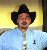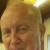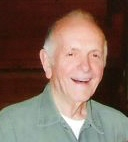## Programming quiz – Excel, VB etc.Tags:
C
COBOL
Microsoft Excel
VB
Recently heard an interesting quiz and thought it will be fun to see the solution done programmatically. The quiz is as follows: A person bought an old car with over 170,000 miles on it. The odometer is a digital device with 6 digits. The odometer shows only full digits – no decimals. One day as the person was ready to leave home for work he noticed the odometer reading is a Palindrome (i.e. the digits are same when reading from front and back - like 175571). After driving about an hour (during this time he also stopped for a cup of coffee on the way) the person reached office. Surprisingly he noticed that the odometer reading is again a palindrome. The question is: How far is the home from the office?

I will admit I did not get the answer by programming – bur rather by trail and error and by intuition. I am trying to solve in Excel but have not finished. I have a good logic for COBOL – but do not have access to COBOL compiler. Will post the answer after hearing from expert readers. Thanks SbElectric

Software/Hardware used:
VB, Excel, C, COBOL

Thanks. We'll let you know when a new response is added.

First I created a function to take three digits and turn them into a six digit palindrome:

Public Function palin(numb As Integer) As Long
palin = 100001 * Mid(numb, 1, 1) + 10010 * Mid(numb, 2, 1) + 1100 * Mid(numb, 3, 1)
End Function

Then I created a sub with a double loop to start from 170 up to 999 in the outer loop (counter i) and i+1 up to 999 in the inner loop. It printed ou the results any time the difference between the palindromes is less than 60 (practical maximum distance to office).

Public Sub main()
Dim i As Integer
Dim j As Integer
Dim MaxNumb As Integer
MaxNumb = 999
For i = 170 To MaxNumb
For j = i + 1 To MaxNumb
If palin(j) – palin(i) < 60 Then
Debug.Print j, i, palin(j), palin(i), palin(j) – palin(i)
End If
Next j
Next i
End Sub

Results are that it is 11 miles from 1999991 to 200002, from 299992 to 300003, etc all the way up to 899998 to 900009. And those are the only results in a reasonable distance to travel in one hour.

## Discuss This Question: 11 Replies

There was an error processing your information. Please try again later.
Thanks. We'll let you know when a new response is added.
Send me notifications when members answer or reply to this question.
•Interesting :-) . Will surely look into it later.
report
•This is very interesting, I've played with it too and it's really above my logic, but I followed what I've seen here and was impressed. Very interesting. Thanks for the question and the logic.
report
• Kudos to Tlsanders1 excellent analysis & programming logic. Yes, the answer is 11. I specially liked the way you set up the palindrome format – it is an interesting concept. By the way, did you realize that the answer will be the same (11 miles) if the odometer is 5 digit or 4 digit? It will be 10 miles if it is a 3 digit device. Here is sample of my pseudo code in COBOL. 01 Num Pic 9(6) 01 Digits redefines Num Pic X occurs 6 times. Now I will set up a Do loop from I = 170000 to 99999 incrementing by 1. Num = I and then compare If Digits (1) = Digits (6) and Digits (2) = Digits (5) and Digits (3) = Digits (4) If True then we found first palindrome. I will place this Do loop under another Loop (J=I + 1 to 99999 then compare the difference to be under 60.. Just like you have done - yours is more elegant! I would like to see a solution via EXCEL. Any EXCEL experts taking up? In Excel is it possible to refer to a subset of a number (like we do in COBOL with occurs clause) without VB programming? Cordially SbElectric
report
•Trivial solution in Excel (but ugly) Fill one column (A) with values from 170 to 999 (e.g. A1 = 170, A2 = A1 + 1, etc.). Fill next column (B) with the palindromic value (Tlsanders1's expression works fine). Fill next column (C) with B2-B1, B3-B2, etc. (fill down works good). Filter on column C for values < 60. You can also write the function in VisualBasic in Excel and return the first value found (or return a string containing the concatenated values found where the value is less than a specific threshold). Of course, if the person lives in Germany and commutes on the Autobahn in their Bugatti Veyron (or similar supercar), any other starting value where the middle two digits are 99 will also yield a palindrome after only 110 miles... (grin)
report
•I got a chuckle out of the autobahn additional solution.
report
•Was trying to find a better way to solve it, but gave up after a few minutes. Tlsanders1's solution is probably the most efficient one.
report
•Actually, the most efficient solution could take advantage of a little trick. If you think about the solution for a few minutes, you realize that only palindromes with "xy99yx" are possible starting solutions. Any other values will not propagate the change in the lower two digits to the upper two. Therefore, the loop is really (vb pseudocode): function Palindrome(byval i1 as int, byval i2 as int, byval i3 as int) as long dim L as long L = (i1*100000) + (i2*10000) + (i3*1000) + (i3*100) + (i2*10) + i1 Palindrome = L end function dim palindromestart as long dim palindromeend as long dim diff as long For i = 17 to 98 palindromestart = palindrome(i / 10, i mod 10, 9) palindromeend = palindrome((i+1)/10, (i+1) mod 10, 0) diff = palindromeend - palindromestart if diff < 120 -- or some arbitrary value print palindromestart, diff end if next i i.e. you only need to evaluate 81 possible starting values.
report
• I enjoyed Kccrosser’s both the analysis and comments. Yes, the 2nd minimum distance will be 110 miles and the 3rd minimum 1100.miles. So your comments on driving a Bugatti was very appropriate. Did you hear about the “million dollar car crash” involving Bentley, Aston-Martin, Porsche 911, Mercedes S class, Ferrari? No... it was not on an autobahn but on an innocuous road in Monte Carlo! Here is a link... enjoy http://money.msn.com/saving-money-tips/post.aspx?post=64e05a5c-d295-4715-9a86-5046c25970a0 with best regards SbElectric
report
• [...] Programming quiz - Excel, VB, etc. [...]
••